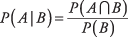## Conditional Probability

Sometimes you have more information than simply total outcomes and favorable outcomes; hence, you are able to make more informed judgments regarding probabilities. For example, suppose you know the following information: In a particular village, there are 60 women and 40 men. Twenty of those women are 70 years of age or older; five of the men are 70 years of age or older (see Table 1).What is the probability that a person selected at random in that town will be a woman? Because women constitute 60 percent of the total population, the probability is 0.60.

What is the probability that a person 70 years of age or older, selected at random, will be a woman? This question is different because the probability of A (being a woman) given B (the person in question being 70 years of age or older) is now conditional upon B (being 70 years of age or older). Because women number 20 out of the 25 people in the 70‐or‐older group, the probability of this latter question is, or 0.80.

Conditional probability is found using this formula:which is read: The probability of A given B equals the probability of A and B divided by the probability of B. The vertical bar in the expression A|B is read given that or given.